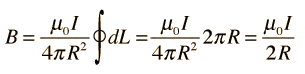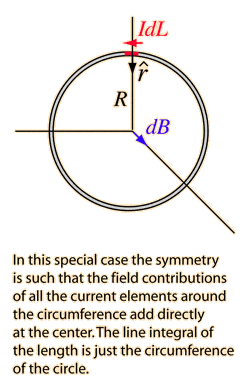# Current Loop Generating a Magnetic Field

• Wilfrid Somogyi
In summary, the magnetic field strength caused by a current loop is governed by the Biot-Savart law. To get the equation for the magnetic field at any point in space, one must take the observation point at the center and integrate around the loop.

#### Wilfrid Somogyi

I'm trying to understand how to calculate the strength of the magnetic field generated by a current loop.

What I know so far is:
• When a current moves around a loop of wire it generates a magnetic field which looks a bit like that of a solenoid, only not so concentrated through the center of the loop.
• The magnetic field at the center of the current loop is calculated by:• I understand what each of the terms mean except the term L and the term r (with the ^ above).
What I'd like to know is:
• How is this equation derived?
• What are the terms I don't recognise?
• Is there a way to calculate the magnetic field strength at any point on the plane that the loop lies in?

Magnetic field strength caused by an elemental current ##I \times dL## is governed by Biot-Savart law $$d\vec{B} = \frac{\mu_0}{4\pi} \frac{Id\vec{L}\times\vec{r}}{r^3}$$. Therefore dL and r (with hat) are elemental source and unit position vector of observation point relative to the elemental source, respectively. To get that equation just take the observation point at the center and integrate around the loop. With Biot-Savart law in hand surely it's possible to calculate magnetic field at any point in space.

blue_leaf77 said:
Magnetic field strength caused by an elemental current ##I \times dL## is governed by Biot-Savart law $$d\vec{B} = \frac{\mu_0}{4\pi} \frac{Id\vec{L}\times\vec{r}}{r^3}$$. Therefore dL and r (with hat) are elemental source and unit position vector of observation point relative to the elemental source, respectively. To get that equation just take the observation point at the center and integrate around the loop. With Biot-Savart law in hand surely it's possible to calculate magnetic field at any point in space.

Thanks, I got most of that, but what do you mean by elemental source? (I'm only an A-level student you see)

The source of magnetic field in our case is a loop in which some currents circulating. If you divide that loop into infinitesimal length (mathematically the length of such object is the limit toward zero), you will get elemental length/source. This is calculus stuff, if you sum infinitesimal elements whose length approaches zero you will end up with integral.
So if one were to picture this problem physically, he must think that the magnetic field at certain point in space must be the sum of magnetic field generated by all existing elemental sources. And again sum of elemental sources forms integral.

Right. So in this case the integral S dL (apologies I have no integral sign on my phone) in the equation just becomes L? I'm assuming that as there is thing between the integral and and dL, so the one has been factored out? In which case why not just represent it as L? Or is it not simply the integral of 1 with respect to L?

if your observation point is in the center and you calculated the cross product correctly you should find that the integrand is axially symmetric.
Try to work it out, it's not that hard.

Will do, thanks for all the help!

## 1. What is a current loop generating a magnetic field?

A current loop generating a magnetic field is a concept in physics where an electric current flowing through a loop of wire creates a magnetic field around the loop. This magnetic field is perpendicular to the direction of the current and is strongest at the center of the loop.

## 2. How does a current loop generate a magnetic field?

When an electric current flows through a wire, it creates a circular magnetic field around the wire. When the wire is formed into a loop, the magnetic field lines become concentrated in the center of the loop, creating a stronger magnetic field. This is due to the combined effect of the individual magnetic fields created by each segment of the wire.

## 3. What are the applications of a current loop generating a magnetic field?

Current loops generating magnetic fields have various applications in everyday life, such as in electric motors, generators, and transformers. They are also used in scientific research, such as in particle accelerators and magnetic resonance imaging (MRI) machines.

## 4. How does the strength of the magnetic field vary with a current loop?

The strength of the magnetic field generated by a current loop depends on several factors, including the current flowing through the loop, the number of turns in the loop, and the distance from the loop. Generally, increasing the current or the number of turns in the loop will result in a stronger magnetic field.

## 5. Can the direction of the magnetic field be changed by changing the direction of the current in the loop?

Yes, the direction of the magnetic field can be changed by changing the direction of the current in the loop. This is known as the right-hand rule, where if you point your thumb in the direction of the current, your fingers will curl in the direction of the magnetic field lines.